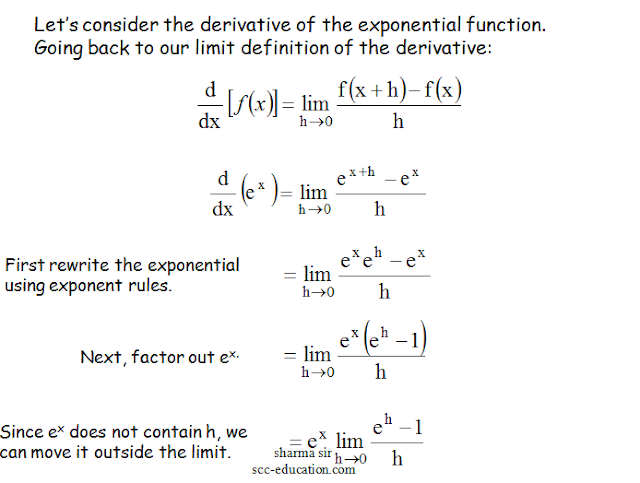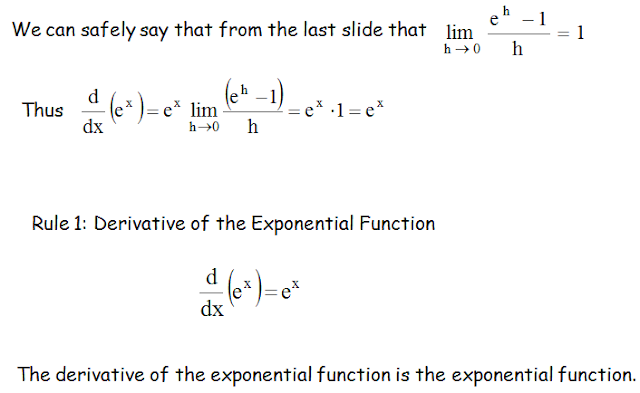# SCC Education

## Differentiation of Exponential Functions

Differentiation of Exponential FunctionsSeries-formulas
The-binomial-theorem
Analytic-trigonometry-double-angle-half angle
solved-problems-on-limits-and-continuity
geometric-progressions
Basic-point-formulas-distance-midpoint
Two-point-form-normal-form parametric
limit-questions
set-relation-function-part1
permutation-combination
straight-lines
Three-diamensional-geometry
Trignometry

hyperbola